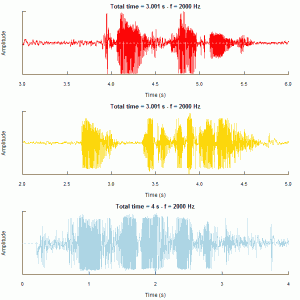# 前言

Surprisingly, penalization methods (e.g., the L0-penalization method) are not optimal for rare/weak signals, even in very simple settings and even with the tuning parameters ideally set。

# 爱的声音1 2 3 4 5 6 7 8 9 10 11 12  library(seewave) library(tuneR) woaini = readMP3("我爱你.mp3") woaini1=resamp(woaini, g = 2000) muqinjie = readMP3("母亲节快乐.mp3") muqinjie1 = resamp(muqinjie, g = 2000) iloveyou = readMP3("i_love_you.mp3") iloveyou1 = resamp(iloveyou, g = 2000) op = par(mfrow = c(3,1),mar = c(4.5,4,2,2)) oscillo(woaini1, f = 2000, from = 3, to = 6,cexlab = 0.75,colwave = "red",title = "妈-我爱你") oscillo(muqinjie1, f = 2000, from = 2, to = 5,cexlab = 0.75,colwave="gold",title = "妈-母亲节快乐") oscillo(iloveyou1, f = 2000, from = 0, to = 4,cexlab = 0.75,colwave="lightblue",title = "i love you so much")• 最上是我用家乡话（信阳罗山话）说的：“妈，我爱你！”（罗山话中，“你”发音类似于“恩”，故振幅小）
• 中间一幅依然用家乡话：“妈，母亲节快乐！”（录得不好，貌似有杂音）
• 最下面的那副是英文：“I love you so much！”

# 看过的“推荐系统”文献

1. 我们最终敲定的模型，参考文献《Pairwise Preference Regression for Cold-start
Recommendation》
。只可惜当时用R写的代码慢的让人自杀，不得不放弃，于是用R整理好数据后，让同学代写C程序（大一时候学的C已经忘得差不多了，真是深感惭愧）。但是谁知道一直有个BUG没有解决，直接导致我们最后参赛结果：0提交！择人不善啊~~以后还是找个靠谱的熟悉矩阵的同学来写C代码吧。模型思想：根据推荐者与被推荐者的features与得分矩阵做拟合，得到推荐与被推荐者features之间权矩阵，然后预测得分。直接了当！模型评价：elegant！思想简约，有数学显式解，对推荐系统的冷启动问题效果应该不错。但是不知道对这次婚介的数据做出来效果是否好，真希望我们能够把那程序完善，去测试一次，了结心愿。
2. 次选模型论文《Hydra: A Hybrid Recommender System》。也很不错，将需要的features信息都能够较好的连接起来，也是通过这个文章，知道了有个对等网络这玩意，也还不错。缺点就是在原基础上扩展的矩阵会很大，特别是对于这次婚介的推荐，SVD做起来消耗估计也会很大，不会太快，效果可能也比较一般。所以对于特征的选取还是得靠前期的数据了解，知道哪些人的特征在此数据中可以省下很多工作。跟这篇文章相关的文章很多，提高精度也罢，改进模型也罢，反正看着挺好，就是不知道用起来如何...sigh~~
3. 《A Singular Value Decomposition Approach Recommendation Systems》这篇文章还是讲SVD的，但是感觉看了那么多论文，感觉这篇文章前面的Introduction介绍推荐系统比较系统全面，如果对推荐系统问题有了较好的定位，我觉得不管做哪些问题，思路都会清晰很多，把握住了问题的关键点，这样结果才会有好的突破。当然这篇文章也详细论述了SVD用在推荐系统的实际意义。
4. 最后再提一个我和小南都认为很不错的论文《Learning User Preferences in Online Dating》，思想很简单，高中生也能不过很能够解决问题。当然这篇文章有个致命的缺陷：数据获取，要能够获得双方发MSG时候的信息。这个实际中估计是是不可能的，所以这篇文章只能提供一个比较好的思路，不能解决实际问题，仅供把玩~~
5. 至于协同过滤的东西，看了挺多，觉得这个东西重要的不是数学，而是他的思想，比如从USER变换到ITEM就可以获得一个很大的突破，真的是让人感觉很畅快。

# 误差论->回归(1)

###竟然发现这篇辛辛苦苦写的文章不见了，1年前我的理解的还是挺正确的 by 2011.9###

1.所有的观测值都可以有误差，其来源可归因与观测者、仪器工具及观测条件。
2.观测误差对称的分布在0 的两侧（排除系统误差）
3.小误差出现的比大误差更频繁。

P.S. 今天老师讲解计量时突然讲不清楚 残差和为何要用$\mathcal{X}^2$检验，我感到很是悲哀，因为这正是误差正态假设的前提下很自然的结果。

# 提高Monte Carlo模拟定积分计算的精度

• 平均值方法是根据弱大数定律证明得来的。构造如此

均方误差

而由$Schwartz$不等式知

,从中便可知 $MC$ 法具有半阶收敛性，且收敛阶不依赖问题的维数，这样在计算高维积分的时候，比较优越。但是收敛速度还是很慢的，是一个比较坏的收敛速度。因此在有其他高效率、高精度的确定型算法时，就不要采用$MC$法，那是在没有更好的算法时更好的抉择，比如超高维积分。
• 由均方误差可知，要提高 $MC$ 法的精确度，那么久必须得增大$N$，减小$VAR(f)$，也就是说，要增加样本量，同时改善抽样方法的选择，这时便有很多方法选择，书中提到了四种方法，我就谈谈我的理解和试验。
1. 分层抽样。核心就是将原来的 $[0,1]$ 区间 $M$ 等分后在各个小区间上分别再以均匀分布抽样 $N/M$ 个点，然后以

理论证明知这样方差减少，具体证明式子较多就不再写，否则就是一本教材的翻版了。
模拟$\int_{0}^{1}exp(-x^2/2)/\sqrt{2pi}dx$,具体实验结果如下

 1 2 3 4 5 6 7  M<-100 N<-1000 A=matrix(as.numeric(gl(M,N/M))-1,ncol=N/M,byrow=T) B=replicate(M,runif(N/M)) x=1/M*t(B)+A/M y=exp(-x^2/2)/sqrt(2*pi) I=mean(y)

结果为0.3413287，可知只取了1000个样本点的情况下，精确度已经十分好了，而一般的均值模拟
达到此精确度则需要$10^7$个点。

2. 对偶变量法。
这是一种特殊的针对于定义域具有一定对称性的函数性质的技巧。基于这样的命题:如果$f(x)$是单调的，则$Cov(f(X),f(1-X))\leq0$,这里$X\sim U[0,1]$。则有

,
$EI_N=I(f)$,
经过证明知 $Var(I_N)\leq \frac{1}{2N}Var(f)$，方差显然减少。

但是这种方法只是对于定义域有一定对称性的函数，如果对一种函数先验了解不多，而且对称性不知，则运用会出现失误，所以具有一定局限性。
模拟$\int_{0}^{1}exp(-x^2/2)/\sqrt{2pi}dx$的代码如下：

 1 2 3  a=runif(10^6) y=exp(-a^2/2)/sqrt(2*pi)+exp(-(1-a)^2/2)/sqrt(2*pi) b=sum(y)/(2*10^6)

结果为 0.3413487，精确度也得到了提高。

3. 重要性抽样：核心就是构造一个新的积分

其中$p(x)$$[0,1]$的概率密度，$\int_{0}^{1}p(x)dx=1$,$p(x)\geq 0$。这样得到类似于最初最简单的那种均值法的新的形式积分近似$I(f)\approx 1/N\sum_{i=1}^{1}f(Y_i)/p(Y_i)$$Y_i$为遵循p(y)的独立同分布随机变量(i.i.d),而之所以这样构造，可以根据这幅图来理解，$\int_{0}^{a}+\int_{b}^{1}f(x)dx$$I(f)$ 很少比例(即图像的边缘部分)，尽可能的不让随机点去计算这些值，这样的“拟合”积分较好（面积）,否则很多的随机点在 $[a,b]$ 外，为计算小比例的东西做贡献，这样必定精度较差，所以我们要期望尽可能的值出现在峰值处。我们称其为“重要性”抽样。理论分析，

,

.
只要选取适当的 $p(y)$ 就可以使方差减少。
当然不可能是$Var(f/p)=0$，这在图的模拟便可知，$p(y)$不可能与$f(x)$相合的同时，还有$\int_{0}^{1}p(x)dx=1$。但是$p$难以选择，需要对问题先验有了一定的了解程度才能适当选取。比如对于正态分布求$\int_{0}^{1}exp(-x^2/2)/\sqrt(2pi)dx$，我不太好选$p$，就没有做相关的模拟了。
4. 控制变量法。
核心也是构造，数学式子$I_N(f)=i/N\sum_{i=1}^{N}(f-g)(X_i)+I(g)$.
需要$I(g)$已知，但是这是同重要性抽样一样，都不知道，也需要对问题先验有了一定的了解程度，才能好的选择，使$I(g)$已知,并且有$Var(f-g)\leq Var(f)$。相关模拟我也没有再做